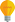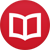# Indefinite integration without variables

by Jakub MarianTip: See my list of theMost Common Mistakes in English. It will teach you how to avoid mis­takes with com­mas, pre­pos­i­tions, ir­reg­u­lar verbs, and much more.

In this article, I’ll assume you have already read my article on differentiation without variables. Just as a quick reminder: $\iota$ denotes the identity function $\iota: ℝ → ℝ$, $\iota(x) = x$, composition of functions is denoted either $f[g]$ or $f ∘ g$, and a function like $f(x) = x^2$ is denoted $f = \iota^2$.

Unlike the traditional approach where indefinite integration is understood as a vague symbolical process with expressions, our definition of indefinite integration will be quite straightforward:

$$∫f = \{g \mid g’ = f\}$$

Using words: $∫f$ is the set of all functions whose derivative is equal to $f$. The question arises of how to denote elementary integrals, e.g. $∫\cos$. Traditionally, one would write $∫\cos(x)dx = \sin(x) + c$. Of course, we could write $∫ {\cos} = \{{\sin}+c\mid c ∈ ℝ\}$, but that’s impractical. A much better way is to define:

$$C = \{c: ℝ → ℝ\mid c\text{ constant}\}$$

(that is, $C$ is the set of all constant functions on $ℝ$). Using it, we can write, for example

$$∫{\cos} = {\sin}+C.$$

I believe it shouldn’t be a problem for the reader to translate the classical tables of integrals into our new notation, e.g.

\begin{align*} ∫ {\sin} &= -{\cos}+C, \\ ∫ ÷{1}{\iota} &= {\log} +C \ \text{ on }(0,∞), \\ ∫ ÷{1}{1+\iota^2} &= {\arctan} + C. \end{align*}

Apart from simple algebraic manipulation, the first method for solving integrals most people have learned was integration by parts. It stays virtually the same in our new notation as in the traditional notation:

$$∫fg’ = fg - ∫ f’g.$$

For example:

$$∫{\iota\sin} = \iota({-\cos})-∫(-{\cos}) = -{\iota\cos}+{\sin}+C.$$

## Integration by substitution

Let’s not beat around the bush with other examples and go directly to the most prominent integration method: integration by substitution. First, a little conventionlet $F$ be a set of functions and $g$ a function. By $F[g]$ or $F∘g$ we mean:

$$F[g] = F ∘ g = \{f ∘ g\mid f ∈ F\}.$$

In other words, we define composition with a set of functions pointwise. Just to give a concrete example: $\{\sin,\cos\}∘{\log} = \{{\sin} ∘ {\log},{\cos}∘{\log}\}$. It is easy to notice the following identity:

$$(f+C)[g] = f[g] + C$$ where $C$, as before, is the set of all constant functions on $ℝ$ (since a constant composed with another function remains a constant). Thanks to it, it is also easy to see yet another identity: $$∫ f[g]g’ = \l(∫ f\r)[g]$$

How to see it?

1. If you differentiate any function in $∫ f[g]g’$, you get $f[g]g’$.
2. All functions in $\l(∫ f\r)[g]$ are of the form $F[g]$ where $F’ = f$. Hence the derivative of any function in $\l(∫ f\r)[g]$ is equal to $(F[g])’ = f[g]g’$.
3. Since the derivatives are equal and both sides already contain all constants, the sets are equal.

And that’s it. This is the integration by “substitution”; we just don’t need any substitution at all. We only have to write the function in a certain form ($f[g]g’$) and then take $g$ out of the integral. For example, what would be traditionally written as:

$$∫ \sin^2(x)\cos(x)dx = \l.∫ t^2 \,dt\,\r|_{t=\sin(x)} = \l.÷{t^3}{3}\r|_{t=\sin(x)} + c = ÷{\sin^3(x)}{3}+c$$

becomes

$$∫ \sin^2 \cos = ∫ \iota^2[\sin]\cos = \l(∫ \iota^2\r)[\sin] = ÷{\iota^3}{3}[\sin] + C = ÷{\sin^3}{3} + C$$

Of course, in practice, we wouldn’t write the first and the third step, but just the ability to write it is of great pedagogical value. The notation without variables is completely rigorous; each expression is well defined as either a function or a set of functions, and each step is an obvious instance of a general rule.

## Separation of variables

Another nice example of how this approach can be used is formalization of the intuitive solution of differential equations by separation of variables. Traditionally, you do the following:

\begin{align*} y’ &= f(x)g(y) \\ ÷{dy}{dx} &= f(x)g(y) \\ ÷{dy}{g(y)} &= f(x)dx \\ ∫ ÷{dy}{g(y)} &= ∫ f(x)dx \end{align*}

Of course, from the mathematical point of view, this is nonsense. Since we are working with indefinite integration, the last step cannot be formalized in any way, even using differential forms. Nevertheless, if you integrate both sides and express $y$ in terms of $x$, you get a correct solution.

Why is this so? The incorrect intuitive method closely mimics the rigorous mathematical one, which is as follows. Let $f$ and $g$ be given functions ($g$ non-zero) and $y$ a function such that:

$$y’ = fg[y]$$

then

\begin{align*} ÷{y’}{g[y]} &= f \\ ÷{1}{g}\![y]\,y’ &= f \\ ∫ ÷{1}{g}\![y]\,y’ &= ∫ f \\ \l(∫ ÷{1}{g}\r)[y] &= ∫ f \end{align*}

We simply used the substitution rule in the last step. Quite elegant, isn’t it?

By the way, I have written several educational ebooks. If you get a copy, you can learn new things and support this website at the same time—why don’t you check them out?